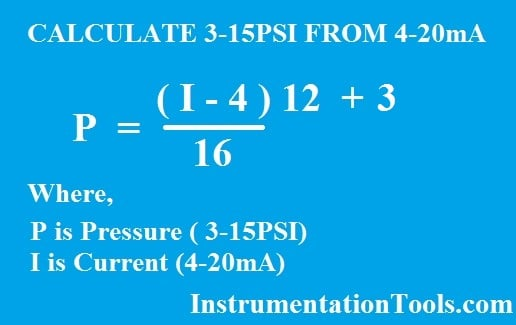# Formula to Calculate 3-15psi from 4-20mA Current

The amount of psi can also be calculated from the amount of the milliamp signal, or one can calculate the amount of the milliamp signal when the amount of psi is known. Each of these signals has an offset and a span that must be taken into account for the calculation.

The offset for the milliamp signal is 4 mA, and the span is 16 mA. The span is determined by subtracting the lower value from the higher value (20 mA – 4 mA). The offset for the psi signal is 3 psi, and the span is 12 psi (15 psi – 3 psi).

The formula for converting between these two systems is shown in the following equation. The milliamp signal is identified as “I” and the pressure signal is identified as “P”. When one is converting from one to the other, either “I” or “P” will become a known value in the formula.

The value 4 in the left side of the equation represents the 4 mA offset, and the value 16 represents the 16 mA span. The value 3 in the right side of the formula represents the pressure offset, and the value 12 represents the 12 psi span.

### (I – 4)/16 = (P – 3)/12

The formula can be further simplified as shown in below figureExample:

#### Calculate the equivalent amount of air pressure for a current signal of 17.5mA ?

P = ((I – 4)/16) x (12) + 3

P = ((17.5 – 4)/16) x (12) + 3

P = 13.125 psi

#### Articles You May Like :

Instrument Technician Questions

Process Variable from 4-20mA

PV to Percentage Conversion

4-20 mA to 0-100% Conversion

4-20mA Graphical Calculations

Be the first to get exclusive content straight to your email.
We promise not to spam you. You can unsubscribe at any time.

### 9 thoughts on “Formula to Calculate 3-15psi from 4-20mA Current”

1.very nice and so easy for understood.

2.mai to mureed to gaya is side ka thanks to all

3.hi, its easy to understand, thanks,,,..! i need one more help . if sensor outputs 4-20mA then controller takes in it and converts it to counts lets say 162 it displays. how can i convert to mA and then to pressure in bar please advice.

•4.Very nice and usefull app

5.use full app

6.plz post the derivation of formula that how to calculate the formula

•7.